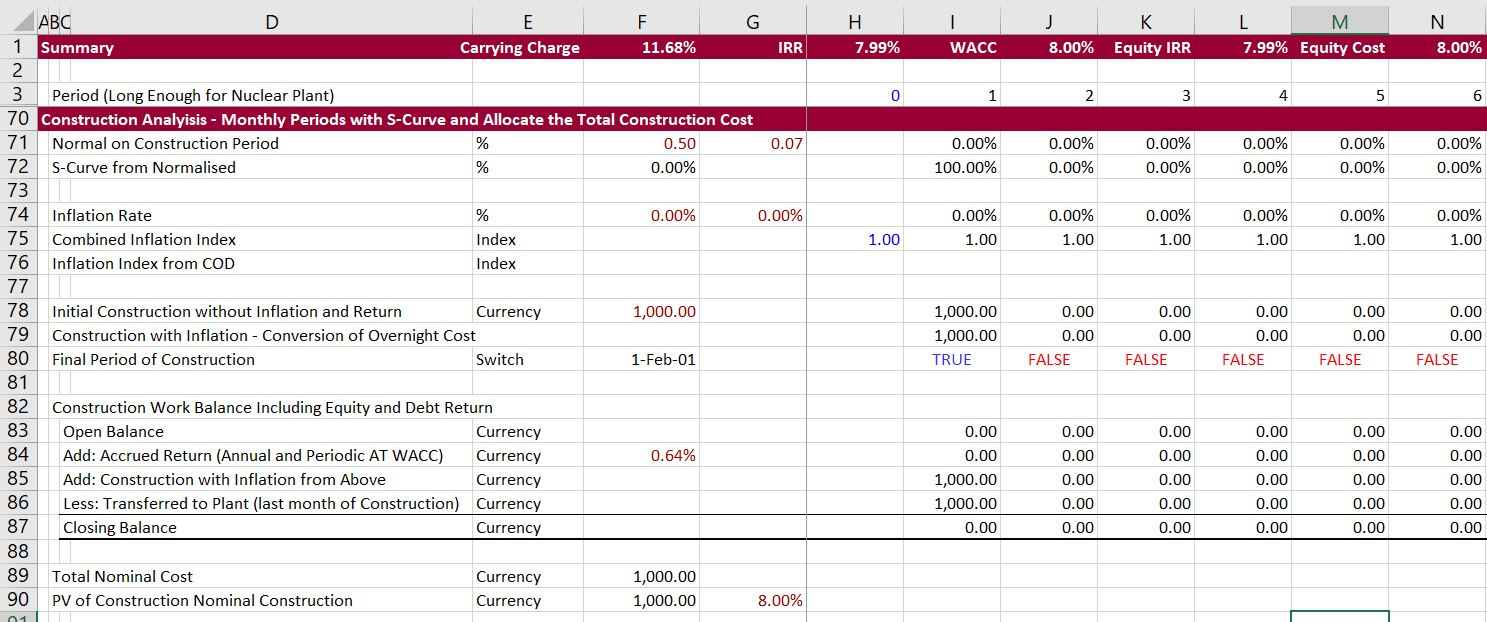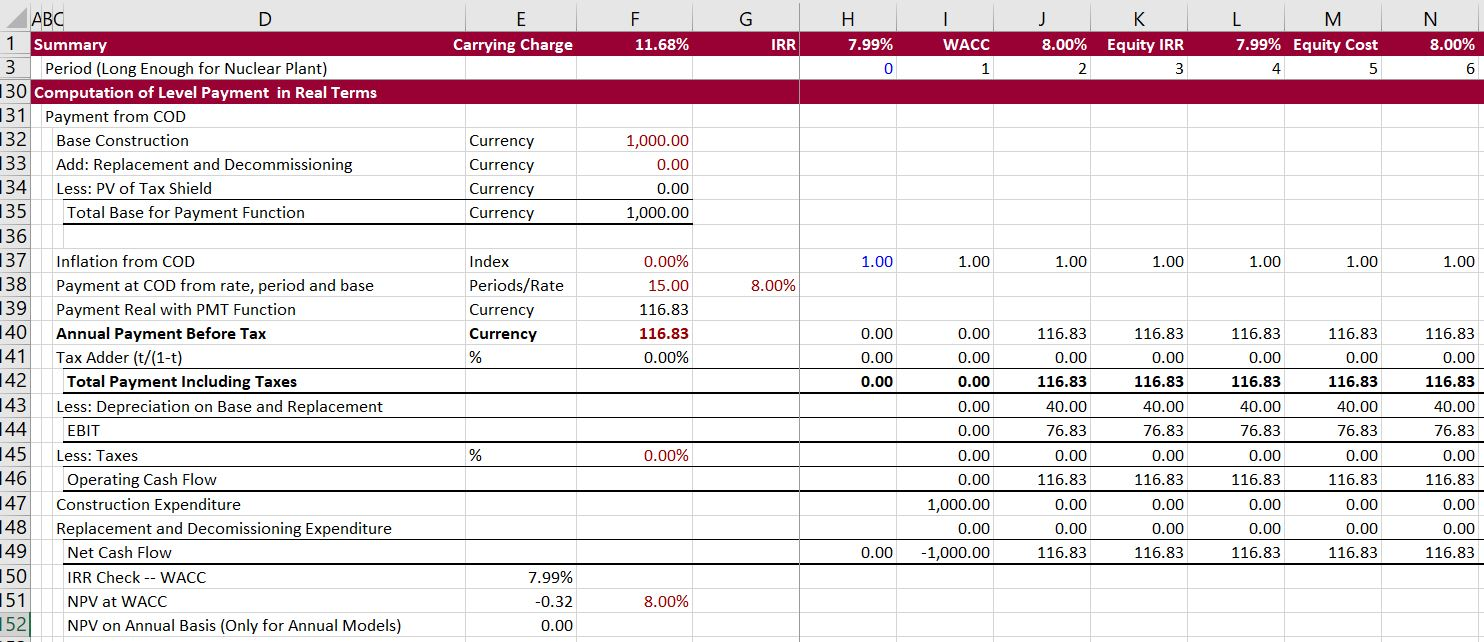# Carrying Charges Part 1 — Fundamentals

To begin the carrying charge discussion, you can think about a situation with no taxes, no inflaton, no debt, no decommissioning, no degradation and a single construction period.  In this case the carrying charge can be computed using the PMT function in excel. The PMT function gives a flat amount that is enough to pay the present value of an investment. The function is often used to compute the mortgage that is paid on a loan.  If the interest rate is zero in the PMT function, the result is simply one divided by the life of the investment.

This lesson set works through calculation of the carrying charges that are used for computing levelised cost of investment. You may think the carrying charge rate is some kind of esoteric concept that is not useful in your day to day work. If you go through this lesson set you will see that the finance, modelling and economic concepts in carrying charge rate analysis are closely related to both project finance and corporate finance.

The carrying charge is a percentage that converts a one time cost that is sometimes called the overnight cost into a level annual cost. (The level cost should be expressed in real terms (i.e. without inflation in constant currency). The set of pages involves using a single capital investment cost and evaluating each of the factors that drive the the conversion of the capital cost into an amount that must be recovered on an annual basis. You can think of this as the amount of revenues necessary to provide a given return to investors.

## Reconciliation of Simple Case

I use the very simple case to introduce you to what I think is a very important file on the website.  This file computes carrying charges using different financing, tax, inflation, tax, construction and decommissioning assumptions.  To demonstrate this case I begin with the simple case and work through the various components of the carrying charge analysis. The comprehensive carrying charge file can be downloaded below.

The first part of the carrying charge file includes the various assumptions that go into computing a level carrying charge. The top line show the outputs from the program including the carrying charge rate and verification that the input cost of capital is produced by a project finance model. In the simple case a rate of return of 8% is assumed along with an asset life of 15.  The carrying charge is not 8%, but a higher value of 11.68% because the retun of the investment as well as the return on the investment must be included in the price.  You can verify this with the PMT function — PMT(8%,15,-1).  The cost of capital assumptions and the outputs from the carrying charge file are shown below.The second part of the carrying charge calculation shows the analysis of construction.  In the simple case I have assumed an immediate overnight construction so that the present value of the construction is equal to the the amount spent on construction.  In presenting capital costs, a so-called over-night assumption is often used.  The excerpt below shows that alternative S-curves with different construction periods and interest rates during construction can be used.  A longer construction period increases the carrying charge rate.  For now, you can see that the assumed construction is equal to the present value of construction that is used in the PMT formula.The third part of the carrying charge spreadsheet moves to replacement cost and decommissioning cost.  Some investments require partial replacement during their life (for example, the inverter for a solar facility or much of a battery).  Decommission costs or residual value should be accounted for in the levelized cost and brought back to the beginning of the analysis. The replacement cost and the decommissioning cost can be brought all the way forward to the end of the construction period in net present value. Once computed, the amount is added to the construction cost for computing the carrying charge rate.  The section on computing relacement cost and decommissioning cost is illustrated in the excerpt below.The fourth part of the carrying charge adjusts the cost for the value of the tax shield.  In computing the carrying charge rate for levelising costs, the present value of the tax shield is broght to the end of the construction period as with the relpacement cost and the decommissioning cost. The reduction in the net cost of the assets is offset by the tax effect of the payment function which is grossed up for taxes using the formula t/(1-t).  The tax adjustments which allow for different depreciation methods and lives are illustrated in the exceprt below.The fifth part of the carrying charge calculation computes the level payment with the adjusted cost and the real cost of capital.  The PMT function is used with the real rate and then the inflated payment is shown.  After computing the payment, the cash flow is confirmed using a cash flow calculation.  In the very simple case with no inflation, the IRR on the cash flow is demonstrated to be equal the cost of capital input (there is a slight difference from the XIRR versus the IRR calculation and the 365 day issue).The sixth section computes the carrying charge from dividing the EBITDA that is produced by the payment function by the investment cost.  The investment cost is the overnight cost input into the model.  This calculation which is the key output from the model is presented at the top of the model.The final parts of the carrying charge spreadsheet include a project fiance model with a debt structure, repayment, interest rates, cash outflows for replacement and decommissioning and with taxes.  The idea of this project finance model is to show that the input target IRR is indeed produced in the model and also that the debt to capital structure is maintained.  This project finance model is documented on a separate page.

## Video Explaining Basics of Carrying Chages

There are a series of videos that describe each of the adjustments that you can make to derive the carrying charge and that shows how the carrying charge spreadsheet was derived.

The first video begins by describing the simple PMT function that accounts for rate of return on investment and the asset life.

The second video below includes all of the adjustments.

Other videos that walk through each of the financial issues are listed at the bottom of the page. I hope you see how the videos describe many economic and technical aspects of project finance models and to some extent even corporate models. The videos also explain added features of the generic macros that modify colours, links, and sheet structure.

After completing this lesson set I hope you will understand the following things about carrying charge rates:

1. Overview of why understanding carrying charges is important
2. Definition of Carrying Charge Rates — Annual recovery cost to total cost; EBITDA to gross investment; Amount of annual recovery to carry investment
3. Normal Complications in Computing Carrying Charge Rates — Construction Period, Inflation, Required Return on Equity, Tax Policies, Capital Structure
4. Addition Complications of Carrying Carrying Charge Rate — Replacement Cost, Decommissioning and Deferred Taxes
5. Project Finance versus Traditional Approach to Computing Carrying Charge Rates
6. Necessity to Convert to Real (i.e. constant) Currency – Would Need to Compute Present value of the Inflated Fuel Cost using Nominal Currency
7. Tax Issues with Carrying Charges and the Concept that Recovery of Equity Returns can be Evalutated with the Formula: Recovery = Recovery + Recovery x t/(1-t)
8. Deferred Tax Complications whereby Recovery can be Computed through First Calculating the PV of the Tax Shield and then using this to Compute the Payment
9 Adjusting the Overnight Cost for Construction Periods with Both Inflation and Financing Cost. Note the Financing Cost must Include Equity and Debt
10. Evaluating the Effects of Future Replacement Cost that can Occur at Different Periods
11. Computing the Effects of Debt on the Analysis using a Constant Capital Structure.

There are a series of videos that describe each of the adjustments that you can make to derive the carrying charge. The video begins by describing the simple PMT.

## Importance of Carrying Charges for Solar Power

The video below demonstrates how carrying charge rates and financial terms affect solar power compared with other technologies.  It demonstrates how you can develop different debt terms, use a goal seek to compute prices and then derive the LCOE.  Using the INDEX function, different technologies are compared with different debt terms.